# Unit-1.pptx

10 de May de 2022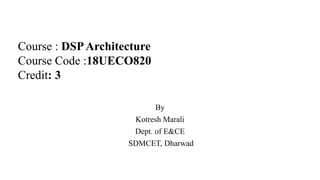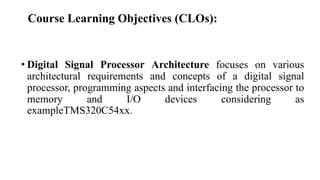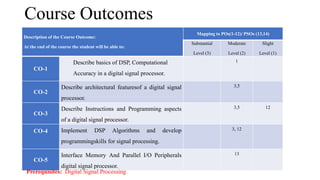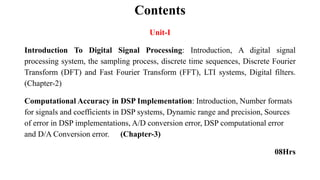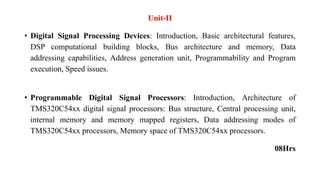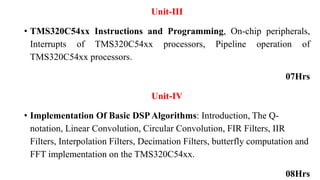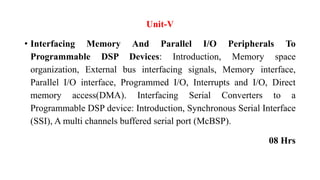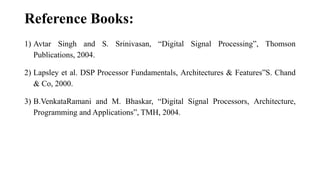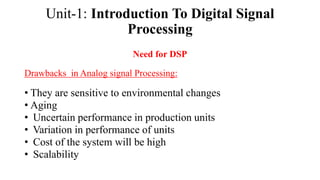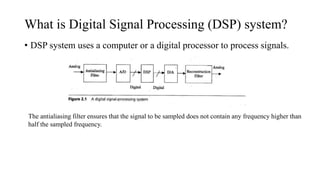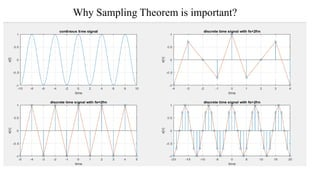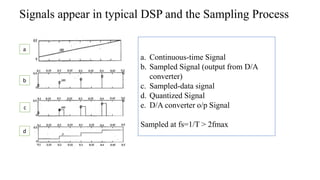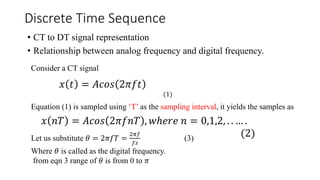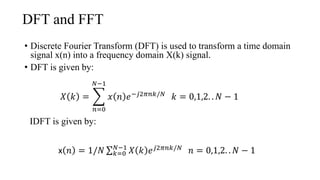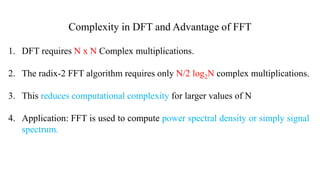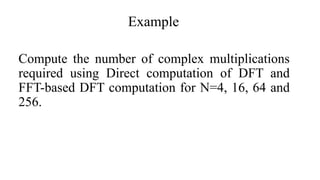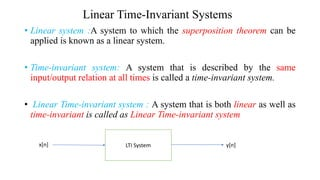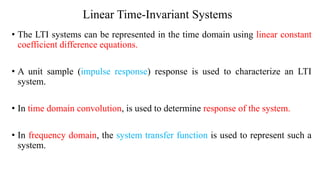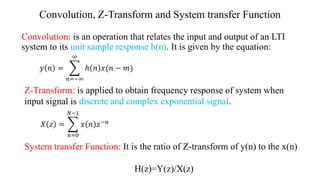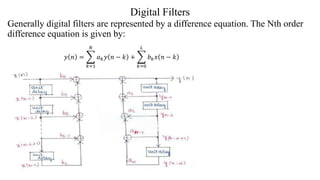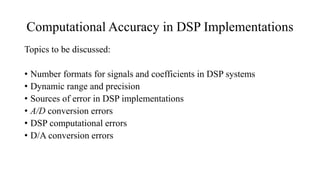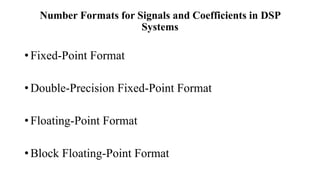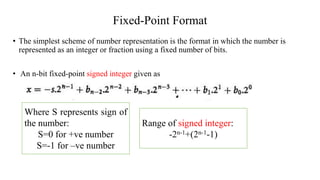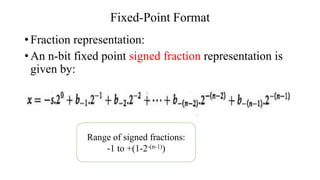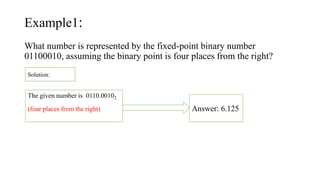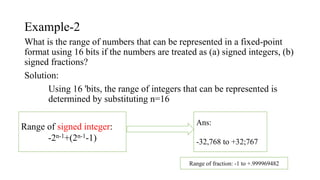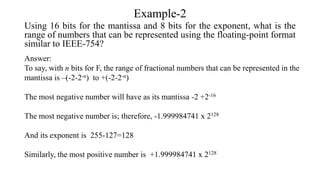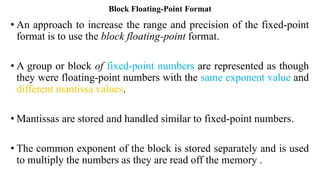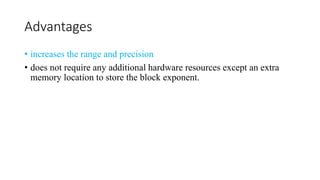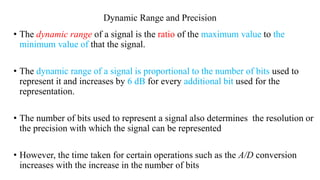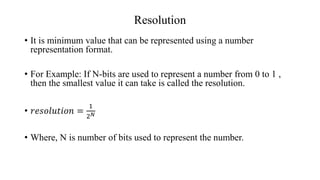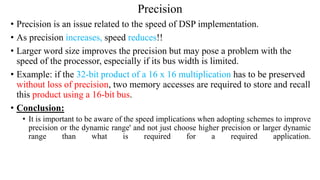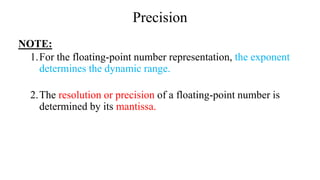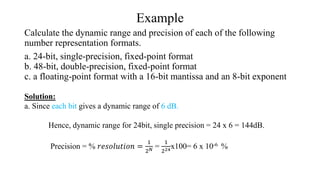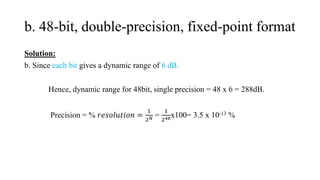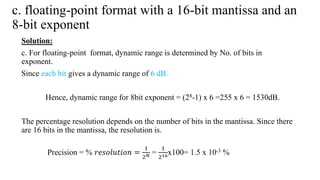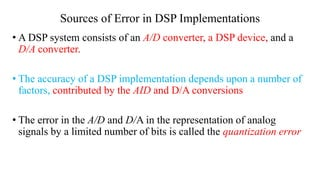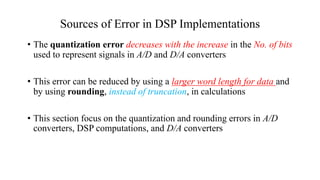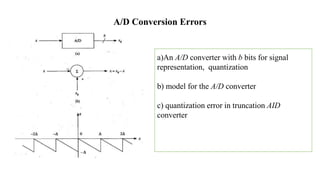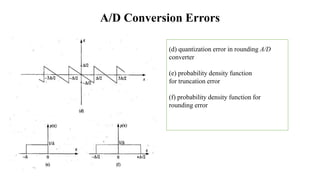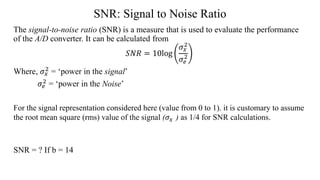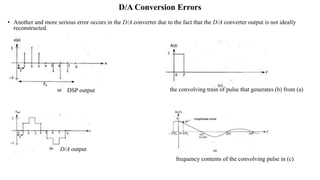1 de 47

### Unit-1.pptx

• 1. By Kotresh Marali Dept. of E&CE SDMCET, Dharwad Course : DSPArchitecture Course Code :18UECO820 Credit: 3
• 2. Course Learning Objectives (CLOs): • Digital Signal Processor Architecture focuses on various architectural requirements and concepts of a digital signal processor, programming aspects and interfacing the processor to memory and I/O devices considering as exampleTMS320C54xx.
• 3. Course Outcomes Description of the Course Outcome: At the end of the course the student will be able to: Mapping to POs(1-12)/ PSOs (13,14) Substantial Level (3) Moderate Level (2) Slight Level (1) CO-1 Describe basics of DSP, Computational Accuracy in a digital signal processor. 1 CO-2 Describe architectural featuresof a digital signal processor. 3,5 CO-3 Describe Instructions and Programming aspects of a digital signal processor. 3,5 12 CO-4 Implement DSP Algorithms and develop programmingskills for signal processing. 3, 12 CO-5 Interface Memory And Parallel I/O Peripherals digital signal processor. 13 Prerequisites: Digital Signal Processing
• 4. Contents Unit-I Introduction To Digital Signal Processing: Introduction, A digital signal processing system, the sampling process, discrete time sequences, Discrete Fourier Transform (DFT) and Fast Fourier Transform (FFT), LTI systems, Digital filters. (Chapter-2) Computational Accuracy in DSP Implementation: Introduction, Number formats for signals and coefficients in DSP systems, Dynamic range and precision, Sources of error in DSP implementations, A/D conversion error, DSP computational error and D/A Conversion error. (Chapter-3) 08Hrs
• 5. Unit-II • Digital Signal Processing Devices: Introduction, Basic architectural features, DSP computational building blocks, Bus architecture and memory, Data addressing capabilities, Address generation unit, Programmability and Program execution, Speed issues. • Programmable Digital Signal Processors: Introduction, Architecture of TMS320C54xx digital signal processors: Bus structure, Central processing unit, internal memory and memory mapped registers, Data addressing modes of TMS320C54xx processors, Memory space of TMS320C54xx processors. 08Hrs
• 6. Unit-III • TMS320C54xx Instructions and Programming, On-chip peripherals, Interrupts of TMS320C54xx processors, Pipeline operation of TMS320C54xx processors. 07Hrs Unit-IV • Implementation Of Basic DSPAlgorithms: Introduction, The Q- notation, Linear Convolution, Circular Convolution, FIR Filters, IIR Filters, Interpolation Filters, Decimation Filters, butterfly computation and FFT implementation on the TMS320C54xx. 08Hrs
• 7. Unit-V • Interfacing Memory And Parallel I/O Peripherals To Programmable DSP Devices: Introduction, Memory space organization, External bus interfacing signals, Memory interface, Parallel I/O interface, Programmed I/O, Interrupts and I/O, Direct memory access(DMA). Interfacing Serial Converters to a Programmable DSP device: Introduction, Synchronous Serial Interface (SSI), A multi channels buffered serial port (McBSP). 08 Hrs
• 8. Reference Books: 1) Avtar Singh and S. Srinivasan, “Digital Signal Processing”, Thomson Publications, 2004. 2) Lapsley et al. DSP Processor Fundamentals, Architectures & Features”S. Chand & Co, 2000. 3) B.VenkataRamani and M. Bhaskar, “Digital Signal Processors, Architecture, Programming and Applications”, TMH, 2004.
• 9. Unit-1: Introduction To Digital Signal Processing Need for DSP Drawbacks in Analog signal Processing: • They are sensitive to environmental changes • Aging • Uncertain performance in production units • Variation in performance of units • Cost of the system will be high • Scalability
• 10. What is Digital Signal Processing (DSP) system? • DSP system uses a computer or a digital processor to process signals. The antialiasing filter ensures that the signal to be sampled does not contain any frequency higher than half the sampled frequency.
• 11. Why Sampling Theorem is important?
• 12. Signals appear in typical DSP and the Sampling Process a. Continuous-time Signal b. Sampled Signal (output from D/A converter) c. Sampled-data signal d. Quantized Signal e. D/A converter o/p Signal Sampled at fs=1/T > 2fmax a b c d
• 13. Discrete Time Sequence • CT to DT signal representation • Relationship between analog frequency and digital frequency. 𝑥 𝑡 = 𝐴𝑐𝑜𝑠 2𝜋𝑓𝑡 (1) Consider a CT signal Equation (1) is sampled using ‘T’ as the sampling interval, it yields the samples as 𝑥 𝑛𝑇 = 𝐴𝑐𝑜𝑠 2𝜋𝑓𝑛𝑇 , 𝑤ℎ𝑒𝑟𝑒 𝑛 = 0,1,2, . . … . (2) Let us substitute 𝜃 = 2𝜋𝑓𝑇 = 2𝜋𝑓 𝑓𝑠 (3) Where 𝜃 is called as the digital frequency. from eqn 3 range of 𝜃 is from 0 to 𝜋
• 14. DFT and FFT • Discrete Fourier Transform (DFT) is used to transform a time domain signal x(n) into a frequency domain X(k) signal. • DFT is given by: 𝑋 𝑘 = 𝑛=0 𝑁−1 𝑥 𝑛 𝑒−𝑗2𝜋𝑛𝑘/𝑁 𝑘 = 0,1,2. . 𝑁 − 1 x 𝑛 = 1/𝑁 𝑘=0 𝑁−1 𝑋 𝑘 𝑒𝑗2𝜋𝑛𝑘/𝑁 𝑛 = 0,1,2. . 𝑁 − 1 IDFT is given by:
• 15. Complexity in DFT and Advantage of FFT 1. DFT requires N x N Complex multiplications. 2. The radix-2 FFT algorithm requires only N/2 log2N complex multiplications. 3. This reduces computational complexity for larger values of N 4. Application: FFT is used to compute power spectral density or simply signal spectrum.
• 16. Compute the number of complex multiplications required using Direct computation of DFT and FFT-based DFT computation for N=4, 16, 64 and 256. Example
• 17. Linear Time-Invariant Systems • Linear system :A system to which the superposition theorem can be applied is known as a linear system. • Time-invariant system: A system that is described by the same input/output relation at all times is called a time-invariant system. • Linear Time-invariant system : A system that is both linear as well as time-invariant is called as Linear Time-invariant system LTI System x[n] y[n]
• 18. • The LTI systems can be represented in the time domain using linear constant coefficient difference equations. • A unit sample (impulse response) response is used to characterize an LTI system. • In time domain convolution, is used to determine response of the system. • In frequency domain, the system transfer function is used to represent such a system. Linear Time-Invariant Systems
• 19. Convolution, Z-Transform and System transfer Function Convolution: is an operation that relates the input and output of an LTI system to its unit sample response h(n). It is given by the equation: 𝑦 𝑛 = 𝑛=−∞ ∞ ℎ 𝑛 𝑥(𝑛 − 𝑚) Z-Transform: is applied to obtain frequency response of system when input signal is discrete and complex exponential signal. 𝑋 𝑧 = 𝑛=0 𝑁−1 𝑥 𝑛 𝑧−𝑛 System transfer Function: It is the ratio of Z-transform of y(n) to the x(n) H(z)=Y(z)/X(z)
• 20. Digital Filters Generally digital filters are represented by a difference equation. The Nth order difference equation is given by: 𝑦 𝑛 = 𝑘=1 𝑁 𝑎𝑘𝑦 𝑛 − 𝑘 + 𝑘=0 𝐿 𝑏𝑘𝑥 𝑛 − 𝑘
• 21. Computational Accuracy in DSP Implementations Topics to be discussed: • Number formats for signals and coefficients in DSP systems • Dynamic range and precision • Sources of error in DSP implementations • A/D conversion errors • DSP computational errors • D/A conversion errors
• 22. Number Formats for Signals and Coefficients in DSP Systems • Fixed-Point Format • Double-Precision Fixed-Point Format • Floating-Point Format • Block Floating-Point Format
• 23. Range of signed integer: -2n-1+(2n-1-1) Fixed-Point Format • The simplest scheme of number representation is the format in which the number is represented as an integer or fraction using a fixed number of bits. • An n-bit fixed-point signed integer given as Where S represents sign of the number: S=0 for +ve number S=-1 for –ve number
• 24. Fixed-Point Format • Fraction representation: • An n-bit fixed point signed fraction representation is given by: Range of signed fractions: -1 to +(1-2-(n-1))
• 25. Example1: What number is represented by the fixed-point binary number 01100010, assuming the binary point is four places from the right? The given number is 0110.00102 (four places from the right) Solution: Answer: 6.125
• 26. Example-2 What is the range of numbers that can be represented in a fixed-point format using 16 bits if the numbers are treated as (a) signed integers, (b) signed fractions? Solution: Using 16 'bits, the range of integers that can be represented is determined by substituting n=16 Range of signed integer: -2n-1+(2n-1-1) Ans: -32,768 to +32;767 Range of fraction: -1 to +.999969482
• 27. Floating-Point Format • A floating-point number is made up of a mantissa Mx and an exponent Ex such that its value x is represented as x = Mx2Ex Figure: IEEE-754 format for floating-point number The value represented by the data format in above Figure is given as x=(-1)s x 2(E - bias) x 1.F F represents the magnitude fraction of the mantissa, Exponent E is an integer. The bias depends upon the bits reserved for the exponent (for 8 bits bias is 127)
• 28. Example-1 Find the decimal equivalent of the floating-point binary number 1011000011100. Assume a format similar to IEEE-754 in which the MSB is the sign bit followed by 4 exponent bits followed by 8 bits for the fractional part. • Solution: • No. is -ve, as the sign bit is 1. • F= 2-4+2-5+2-6 = 0.109375 • E=21+22 = 6 • Thus, the value of the number is x=(-1)s x 2(E - bias) x 1.F • x= -1.109375 X 2(6-7)= -0.5546875
• 29. Example-2 Using 16 bits for the mantissa and 8 bits for the exponent, what is the range of numbers that can be represented using the floating-point format similar to IEEE-754? Answer: To say, with n bits for F, the range of fractional numbers that can be represented in the mantissa is –(-2-2-n) to +(-2-2-n) The most negative number will have as its mantissa -2 +2-16 The most negative number is; therefore, -1.999984741 x 2128 And its exponent is 255-127=128 Similarly, the most positive number is +1.999984741 x 2128
• 30. Block Floating-Point Format • An approach to increase the range and precision of the fixed-point format is to use the block floating-point format. • A group or block of fixed-point numbers are represented as though they were floating-point numbers with the same exponent value and different mantissa values. • Mantissas are stored and handled similar to fixed-point numbers. • The common exponent of the block is stored separately and is used to multiply the numbers as they are read off the memory .
• 31. Advantages • increases the range and precision • does not require any additional hardware resources except an extra memory location to store the block exponent.
• 32. Dynamic Range and Precision • The dynamic range of a signal is the ratio of the maximum value to the minimum value of that the signal. • The dynamic range of a signal is proportional to the number of bits used to represent it and increases by 6 dB for every additional bit used for the representation. • The number of bits used to represent a signal also determines the resolution or the precision with which the signal can be represented • However, the time taken for certain operations such as the A/D conversion increases with the increase in the number of bits
• 33. Resolution • It is minimum value that can be represented using a number representation format. • For Example: If N-bits are used to represent a number from 0 to 1 , then the smallest value it can take is called the resolution. • 𝑟𝑒𝑠𝑜𝑙𝑢𝑡𝑖𝑜𝑛 = 1 2𝑁 • Where, N is number of bits used to represent the number.
• 34. Precision • Precision is an issue related to the speed of DSP implementation. • As precision increases, speed reduces!! • Larger word size improves the precision but may pose a problem with the speed of the processor, especially if its bus width is limited. • Example: if the 32-bit product of a 16 x 16 multiplication has to be preserved without loss of precision, two memory accesses are required to store and recall this product using a 16-bit bus. • Conclusion: • It is important to be aware of the speed implications when adopting schemes to improve precision or the dynamic range' and not just choose higher precision or larger dynamic range than what is required for a required application.
• 35. Precision NOTE: 1.For the floating-point number representation, the exponent determines the dynamic range. 2.The resolution or precision of a floating-point number is determined by its mantissa.
• 36. Example Calculate the dynamic range and precision of each of the following number representation formats. a. 24-bit, single-precision, fixed-point format b. 48-bit, double-precision, fixed-point format c. a floating-point format with a 16-bit mantissa and an 8-bit exponent Solution: a. Since each bit gives a dynamic range of 6 dB. Hence, dynamic range for 24bit, single precision = 24 x 6 = 144dB. Precision = % 𝑟𝑒𝑠𝑜𝑙𝑢𝑡𝑖𝑜𝑛 = 1 2𝑁 = 1 224x100= 6 x 10-6 %
• 37. b. 48-bit, double-precision, fixed-point format Solution: b. Since each bit gives a dynamic range of 6 dB. Hence, dynamic range for 48bit, single precision = 48 x 6 = 288dB. Precision = % 𝑟𝑒𝑠𝑜𝑙𝑢𝑡𝑖𝑜𝑛 = 1 2𝑁 = 1 248x100= 3.5 x 10-13 %
• 38. c. floating-point format with a 16-bit mantissa and an 8-bit exponent Solution: c. For floating-point format, dynamic range is determined by No. of bits in exponent. Since each bit gives a dynamic range of 6 dB. Hence, dynamic range for 8bit exponent = (28-1) x 6 =255 x 6 = 1530dB. The percentage resolution depends on the number of bits in the mantissa. Since there are 16 bits in the mantissa, the resolution is. Precision = % 𝑟𝑒𝑠𝑜𝑙𝑢𝑡𝑖𝑜𝑛 = 1 2𝑁 = 1 216x100= 1.5 x 10-3 %
• 39. Sources of Error in DSP Implementations • A DSP system consists of an A/D converter, a DSP device, and a D/A converter. • The accuracy of a DSP implementation depends upon a number of factors, contributed by the AID and D/A conversions • The error in the A/D and D/A in the representation of analog signals by a limited number of bits is called the quantization error
• 40. • The quantization error decreases with the increase in the No. of bits used to represent signals in A/D and D/A converters • This error can be reduced by using a larger word length for data and by using rounding, instead of truncation, in calculations • This section focus on the quantization and rounding errors in A/D converters, DSP computations, and D/A converters Sources of Error in DSP Implementations
• 41. A/D Conversion Errors a)An A/D converter with b bits for signal representation, quantization b) model for the A/D converter c) quantization error in truncation AID converter
• 42. A/D Conversion Errors (d) quantization error in rounding A/D converter (e) probability density function for truncation error (f) probability density function for rounding error
• 43. SNR: Signal to Noise Ratio The signal-to-noise ratio (SNR) is a measure that is used to evaluate the performance of the A/D converter. It can be calculated from 𝑆𝑁𝑅 = 10log 𝜎𝑥 2 𝜎𝑒 2 Where, 𝜎𝑥 2 = ‘power in the signal’ 𝜎𝑒 2 = ‘power in the Noise’ For the signal representation considered here (value from 0 to 1). it is customary to assume the root mean square (rms) value of the signal (𝜎𝑥 ) as 1/4 for SNR calculations. SNR = ? If b = 14
• 44. D/A Conversion Errors • Another and more serious error occurs in the D/A converter due to the fact that the D/A converter output is not ideally reconstructed. DSP output D/A output the convolving train of pulse that generates (b) from (a) frequency contents of the convolving pulse in (c)